# Texas Go Math Grade 4 Lesson 15.4 Answer Key Customary Units of Liquid Volume

Refer to our Texas Go Math Grade 4 Answer Key Pdf to score good marks in the exams. Test yourself by practicing the problems from Texas Go Math Grade 4 Lesson 15.4 Answer Key Customary Units of Liquid Volume.

## Texas Go Math Grade 4 Lesson 15.4 Answer Key Customary Units of Liquid Volume

Essential Question

How can you convert customary units of liquid volume?
In the US customary system of measurement, the most common units of volume are cups, pints, quarts and gallons

Unlock the Problem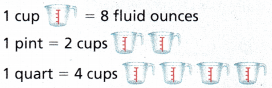Liquid volume is the measure of the space a liquid occupies. Some basic units for measuring liquid volume are gallons, half gallons, quarts, pints, and cups.

The bars below model the relationships among some units of liquid volume. The largest units are gallons. The smallest units are fluid ounces.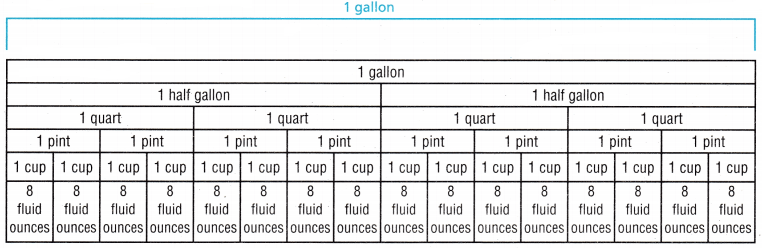Example How does the size of a gallon compare to the size of a quart?

STEP 1 Draw two bars that represent this relationship. One bar should show gallons and the other bar should show quarts.
Red bar represents Gallon
Yellow bar represents quart

STEP 2 Shade 1 gallon on one bar and shade 1 quart on the other bar.
Labeled them as gallon and quart

STEP 3 Compare the size of 1 gallon to the size of 1 quart.
So, 1 gallon is ___________ times as much as 1 quart.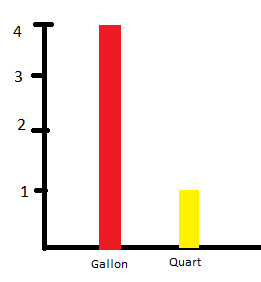Explanation:
1 gallon = 4 quarts
So, 1 gallon is 4 times as much as 1 quart.

Math Talk

Mathematical Processes
Describe the pattern in the units of liquid volume.
The pattern in units are 1 divided to halves
and then to fourths
and then to eigths.

Example 1 Convert larger units to smaller units.
Serena is making 3 gallons of lemonade to sell with her friend. Her friend wants to know how many fluid ounces of lemonade Serena made. How many fluid ounces of lemonade did Serena make?

STEP 1 Use the model on page 537. Find the relationship between gallons and fluid ounces.
1 gallon = __________ cups
1 cup = __________ fluid ounces
1 gallon = __________ cups × __________ fluid ounces
1 gallon = ___________ fluid ounces
1 gallon = 16 cups
1 cup = 8 fluid ounces
1 gallon = 16 cups × 8 fluid ounces
1 gallon = 128 fluid ounces

STEP 2 Make a table that relates gallons and fluid ounces.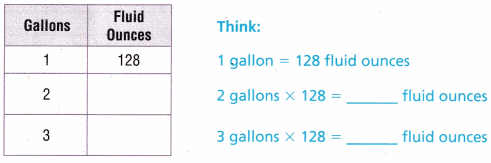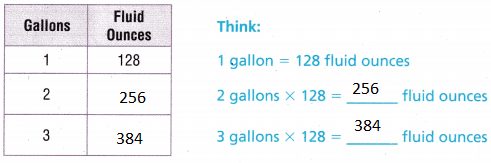Explanation:
384 fluid ounces of lemonade that Serena make

Example 2 Convert smaller units to larger units.

Allison needs to make 36 cups of fruit punch for a party. The recipe she has uses quarts instead of cups. How many quarts of fruit punch does Allison need to make for the party?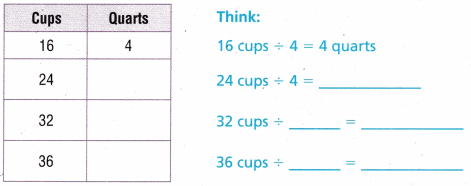So, Allison made __________ quarts of fruit punch.Explanation:
So, Allison made 9 quarts of fruit punch.
Allison need to make for the party 9 quarts.

Share and Show

Question 1.
Compare the size of a quart to the size of a pint. Use a model to help.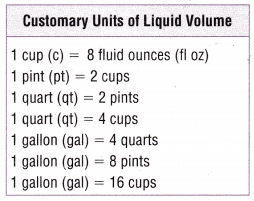1 quart is __________ times as much as __________ pint.Explanation:
1 quart is 2 times as much as 1 pint.

Complete.

Question 2.
4 cups = __________ pints
4 cups = 2 pints
Explanation:
1 pints  = 2 cups
4 ÷ 2 = 2

12 quarts = __________ gallons
12 quarts = 3 gallons
Explanation:
1 gallon = 4 quarts
12 ÷ 4 = 3

Question 4.
6 quarts = __________ cups
6 quarts = 24 cups
Explanation:
1 quart = 4 cups
6 x 4 = 24

Question 5.
20 cups = __________ quarts
20 cups = 5 quarts
Explanation:
1 quart = 4 cups
20 ÷ 4 = 5

Question 6.
3 gallons = __________ pints
3 gallons = 24pints
Explanation:
1 gallon = 8 pints
3 x 8 = 24 pints

Question 7.
16 pints = __________ cups
16 pints = 32 cups
Explanation:
1 pints  = 2 cups
16 x  2 = 32

Math Talk

Mathematical Processes
Explain how the conversion chart above relates to the model in Exercise 1.
The chart shows values of liquids
Explanation:
The measures are given in the chart
with the help of chart we can easily analyze the answer

Problem Solving

Question 8.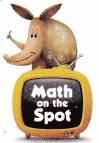H.O.T. Use Representations Multi-Step A soccer team has 25 players. The team’s thermos holds 4 gallons of water. If the thermos is full, is there enough water for each player to have 2 cups? Explain. Make a table to help.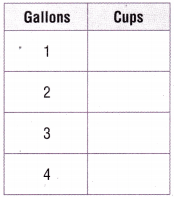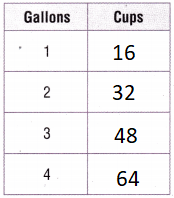Explanation:
1 gallon = 16 cups
25 x 2 = 50
64 – 50 = 14
Yes there will be enough water.

Go Math Grade 4 Volume 2 Customary Units of Liquid Volume Question 9.
H.O.T. Penelope drank 64 fluid ounces of water each day for 7 days.
How many cups of water did Penelope drink during those 7 days? Explain.
8 cups of water that Penelope drink during those 7 days
Explanation:
1 cup = 8 fluid ounces
64 ÷ 8  = 8

Question 10.
A corn plant gives off about 9 gallons of water each month during the growing season. How many pints of water does the corn plant give off each month?
(A) 144 pints .
(B) 36 pints
(C) 72 pints
(D) 18 pints
Explanation:
1 gallon= 8 pints
9 x 8 = 72
72 pints of water that the corn plant give off each month

Question 11.
Ken makes 6 quarts of fruit juice. He serves the juice in glasses that hold 1 cup. How many glasses of fruit juice does Ken serve?
(A) 12 glasses
(B) 24 glasses
(C) 96 glasses
(D) 48 glasses
Explanation:
1 quart = 4 cups
6 x 4 = 24 glasses

Question 12.
Multi-Step Marty’s pitcher holds 64 ounces of water. She drinks 4 cups of water in the morning and 2 cups in the afternoon. How much water is left in the pitcher?
(A) 40 ounces
(B) 52 ounces
(C) 32 ounces
(D) 16 ounces
Explanation:
4 + 2 = 6
1 cup = 8 fluid ounces
6 x 8 = 48
64 – 48 = 16
16 ounces of water is left in the pitcher

TEXAS Test Prep

A pitcher contains 5 quarts of water. How many cups of water does the pitcher contain?
(A) 4 Cups
(B) 10 cups
(C) 20 cups
(D) 40 cups
Explanation:
1 quart = 4 cups
5 x 4 = 20
20 cups of water that the pitcher contains

### Texas Go Math Grade 4 Lesson 15.4 Homework and Practice Answer Key

Complete.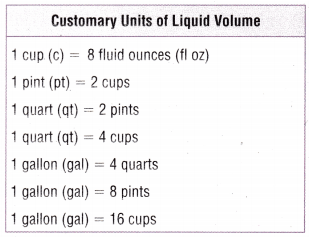Question 1.
5 gallons = _____________ pints
5 gallons = 40 pints
Explanation:
1 gallon = 8 pints
5 x 8 = 40

Question 2.
20 cups = _____________ quarts
20 cups = 5 quarts
Explanation:
1 quart = 4 cups
20 ÷ 4 = 5

Question 3.
3 cups = _____________ fluid ounces
3 cups = 24 fluid ounces
Explanation:
1 cup = 8 fluid ounces
3 x 8 = 24 ounces

Go Math Grade 4 Lesson 15.4 Homework Question 4.
6 pints = _____________ quarts
6 pints = 3 quarts
Explanation:
2 pints = 1 quart
6 ÷ 2 = 3

Question 5.
16 quarts = _____________ gallons
16 quarts = 4 gallons
Explanation:
1 gallon = 4 quarts
16 ÷ 4 = 4

Question 6.
40 fluid ounces = _____________ cups
40 fluid ounces = 5 cups
Explanation:
1 cup = 8 fluid ounces
40 ÷ 8 = 5

Problem Solving

Question 7.
Marina’s mug holds 12 fluid ounces of liquid. She drinks6 mugs of liquid a day. How many cups of liquid does she drink? Explain. Make a table to help you.Explanation:
6 x 12 = 72
9 cups of liquid that she drink

Question 8.
Joshua made 5 gallons of maple syrup. How many pint jars does he need to hold the syrup? Explain.
5 gallons = 40 pints
Explanation:
1 gallon = 8 pints
5 x 8 = 40
40 pint jars that he needs to hold the syrup

Texas Go Math Grade 4 Volume 1 Pdf Lesson 15.4 Question 9.
The label on a bottle of apple juice says it contains 128 fluid ounces. how many quarts of juice are in the bottle? Explain.
128 fluid ounces. = 4 quarts
Explanation:
1 cup = 8 fluid ounces
1 quart = 4 cups
128 ÷ 4 = 32
32 ÷ 8 = 4
4 quarts of juice are in the bottle

Lesson Check

Question 10.
Michael has a pail that holds 5 gallons of water. How many quarts of water does the pail hold?
(A) 40 quarts
(B) 20 quarts
(C) 10 quarts
(D) 15 quarts
Explanation:
1 gallon = 4 quarts
5 x 4 = 20
20 quarts of water that the pail hold

Question 11.
Emily needs 12 cups of fruit juice for a punch recipe. The juice comes in quart bottles. How many bottles of juice should Emily buy?
(A) 6
(B) 4
(C) 2
(D) 3
Explanation:
1 quart = 4 cups
12 ÷  4 = 3
3 bottles of juice that Emily buy

Question 12.
Multi-Step Marsha’s thermos holds 3 pints of tea. She drinks 2 cups of tea on her break and 1 cup of tea for lunch. How many cups of tea are left in the thermos?
(A) 3 cups
(B) 2 cups
(C) 6 cups
(D) 4 cups
Explanation:
1 pint = 2 cups
3 x 2 = 6
2 + 1 = 3 cups
6 – 3 = 3
3 cups of tea left

Question 13.
Multi-Step Each batch of pancakes Arlo makes needs 2 cups of milk. How many batches of pancakes can Arlo make with 2 quarts of milk?
(A) 8
(B) 4
(C) 2
(D) 16
Explanation:
1 quart = 4 cups
2 x 4 = 8
8 ÷  2 = 4
4 batches of pancakes can Arlo make with 2 quarts of milk

Multi-Step Antonia made 64 fluid ounces of jam. How many pint jars can Antonia fill with the jam?
(A) 6
(B) 2
(C) 4
(D) 8
Explanation:
1 pint = 2 cups
1 cup = 8 fluid ounces
64 ÷ 2 = 32
32 ÷ 8 = 4
4 pint jars can Antonia fill with the jam

Question 15.
Multi-Step Peter’s glasses each hold 8 fluid ounces. How many glasses of juice can Peter pour from a bottle that holds 2 quarts?
(A) 8
(B) 4
(C) 16
(D) 12